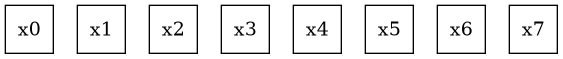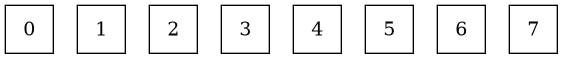# Question

Is there a way to use part of the node ID in label?

In the example below, I’d like to use the last character. The
alternative solution would remove the prefix `x`.

# MWE

``````digraph x {
node [shape="square"]
x0
x1
x2
x3
x4
x5
x6
x7
}
``````

# Have# Want# Notes

Simply removing the prefix `x` in the node name is not a solution for more complex graphs.

I was thinking there might be some syntax that can be combined with `\N` (e.g., `\N[-1]`).

Does this `x5 [label=5]` not work for you - i.e. generate each node’s label explicitly?

If not, no there is nothing in the Graphviz language similar to what you want.
However, the following gvpr (https://www.graphviz.org/pdf/gvpr.1.pdf) one-liner will strip the all alpha characters off each node name & assign the result to the node label. More string manipulation is available. (Note, gvpr uses ksh/bash pattern-matching syntax!)

``````gvpr -c 'N{\$.label=sub(\$.name,"+([a-zA-Z])");}' myfile.gv >myfileNew.gv
``````

Giving:

``````	graph [rankdir=LR];
node [label=""];
edge [style=invis];
subgraph subset {
node [shape=none];
s0	[label=0,
shape=square];
s1	[label=1];
s0 -> s1;
s3	[label=3,
shape=square];
s4	[label=4];
s3 -> s4;
s5	[label=5,
shape=square];
s6	[label=6,
shape=square];
s5 -> s6;
s7	[label=7];
s6 -> s7;
s2	[label=2];
s1 -> s2;
s2 -> s3;
s4 -> s5;
}
subgraph fullset {
node [shape=square];
fs0	[label=0];
fs1	[label=1];
fs0 -> fs1;
fs2	[label=2];
fs1 -> fs2;
fs3	[label=3];
fs2 -> fs3;
fs4	[label=4];
fs3 -> fs4;
fs5	[label=5];
fs4 -> fs5;
fs6	[label=6];
fs5 -> fs6;
fs7	[label=7];
fs6 -> fs7;
}
}
``````

Note that directly manipulating a label value containing escape-string characters is more work. The Graphviz parsers do not show expanded escape-chars until very late in the game - see:

``````dot -Tdot myfile.gv
``````

note that \N is not shown expanded